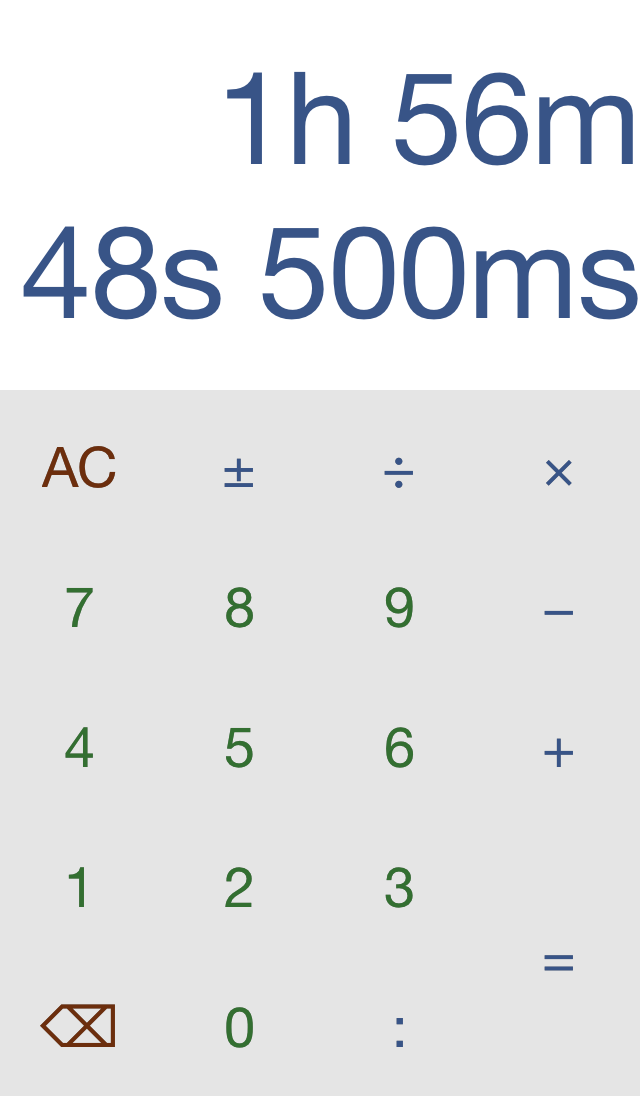Get it? CrunchTime
Crunch time.
A simple, versatile time calculator for \$GET (a button press) (with ads).× Calculate dates, times, years, days, hours, minutes, & seconds.

− Simple design, powerful functionality

÷ Includes addition, subtraction, multiplication, division, and more

+ Works just like you would expect a calculator to work

• This version contains ads
• If I ran a 5k in 20:09, then what was my pace?: 20:09 ÷ 3.1 = 6:30.
• What if I took about 7 seconds off my pace, then how long would it take to complete my workout?: 6:30 - 7 = 6:23 × 3.1 = 19:48.
• If I do something for 35 minutes 12 times, how much total time did it take?: 35:00 × 12 = 7h 00m 00s.
• Answer these, and other time-related questions right now! =

Crunch time with crunchtime.